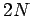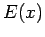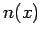Next: Evaluation of electron number Up: Particle-in-cell codes Previous: Normalization scheme

## Solution of electron equations of motion

We can solve the electron equations of motion, (295) and (296), as a set ofcoupled first-order ODEs using the RK4 methods discussed in Sect. 3. However, in order to evaluate the right-hand sides of these equations we need to know the electric fieldat each time-step. We can achieve this by solving Poisson's equation, (298), every time-step. However, in order to determine the source term for this equation we need to calculate the electron number density, which is, of course, a function of the instantaneous electron locations.

Richard Fitzpatrick 2006-03-29